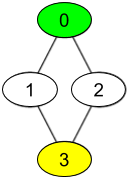1311. Get Watched Videos by Your Friends

There are `n` people, each person has a unique id between `0` and `n-1`. Given the arrays `watchedVideos` and `friends`, where `watchedVideos[i]` and `friends[i]` contain the list of watched videos and the list of friends respectively for the person with `id = i`.

Level 1 of videos are all watched videos by your friends, level 2 of videos are all watched videos by the friends of your friends and so on. In general, the level `k` of videos are all watched videos by people with the shortest path exactly equal to `k` with you. Given your `id` and the `level` of videos, return the list of videos ordered by their frequencies (increasing). For videos with the same frequency order them alphabetically from least to greatest.

Example 1:```Input: watchedVideos = [["A","B"],["C"],["B","C"],["D"]], friends = [[1,2],[0,3],[0,3],[1,2]], id = 0, level = 1
Output: ["B","C"]
Explanation:
You have id = 0 (green color in the figure) and your friends are (yellow color in the figure):
Person with id = 1 -> watchedVideos = ["C"]
Person with id = 2 -> watchedVideos = ["B","C"]
The frequencies of watchedVideos by your friends are:
B -> 1
C -> 2
```

Example 2:```Input: watchedVideos = [["A","B"],["C"],["B","C"],["D"]], friends = [[1,2],[0,3],[0,3],[1,2]], id = 0, level = 2
Output: ["D"]
Explanation:
You have id = 0 (green color in the figure) and the only friend of your friends is the person with id = 3 (yellow color in the figure).
```

Constraints:

• `n == watchedVideos.length == friends.length`
• `2 <= n <= 100`
• `1 <= watchedVideos[i].length <= 100`
• `1 <= watchedVideos[i][j].length <= 8`
• `0 <= friends[i].length < n`
• `0 <= friends[i][j] < n`
• `0 <= id < n`
• `1 <= level < n`
• if `friends[i]` contains `j`, then `friends[j]` contains `i`

1311. Get Watched Videos by Your Friends
``````struct Solution;
use std::collections::HashMap;
use std::collections::VecDeque;

impl Solution {
fn watched_videos_by_friends(
watched_videos: Vec<Vec<String>>,
friends: Vec<Vec<i32>>,
id: i32,
level: i32,
) -> Vec<String> {
let n = watched_videos.len();
let mut visited = vec![false; n];
let mut queue: VecDeque<(usize, i32)> = VecDeque::new();
let id = id as usize;
visited[id] = true;
queue.push_back((id, 0));
let mut freq: HashMap<String, usize> = HashMap::new();
while let Some((u, l)) = queue.pop_front() {
if l < level {
for &friend in &friends[u] {
let v = friend as usize;
if !visited[v] {
visited[v] = true;
queue.push_back((v, l + 1));
}
}
} else {
for video in &watched_videos[u] {
*freq.entry(video.to_string()).or_default() += 1;
}
}
}
let mut pairs: Vec<(usize, String)> = vec![];
for (video, count) in freq {
pairs.push((count, video));
}
pairs.sort_unstable();
pairs.into_iter().map(|p| p.1).collect()
}
}

#[test]
fn test() {
let watched_videos = vec_vec_string![["A", "B"], ["C"], ["B", "C"], ["D"]];
let friends = vec_vec_i32![[1, 2], [0, 3], [0, 3], [1, 2]];
let id = 0;
let level = 1;
let res = vec_string!["B", "C"];
assert_eq!(
Solution::watched_videos_by_friends(watched_videos, friends, id, level),
res
);
let watched_videos = vec_vec_string![["A", "B"], ["C"], ["B", "C"], ["D"]];
let friends = vec_vec_i32![[1, 2], [0, 3], [0, 3], [1, 2]];
let id = 0;
let level = 2;
let res = vec_string!["D"];
assert_eq!(
Solution::watched_videos_by_friends(watched_videos, friends, id, level),
res
);
}
``````